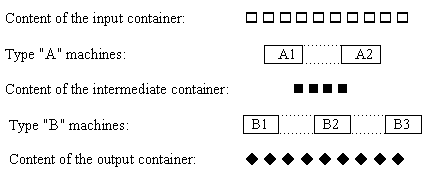## A Game

Consider the following two-player game. The game board consists of a sequence of positive integers. The two players move alternately. When a player moves, he selects a number from either the left or the right end of the sequence. The selected number is deleted from the board. The game is over when all numbers have been selected. The first player wins if the sum of the numbers he has selected is at least as much as selected by the second player. The second player plays his best.

The first player starts the game.

If the board initially contains an even number of elements, then the first player has a winning strategy. You are to write a program that implements the strategy of the first player to win the game. The second player's response is provided by a given computer program. The two players communicate with three procedures of the module Play that is made available to you. These procedures are StartGame, MyMove and YourMove. The first player should initiate the game by executing the parameterless procedure StartGame. If the first player selects a number from the left end, he executes the procedure MyMove('L'). Similarly, executing the instruction MyMove('R') sends a message to the second player indicating that the first player has selected a number from the right end. The second player, i.e. the machine moves immediately, and the first player can learn this move by executing the instruction YourMove(C), where C is a character type variable(in C/C++ you write this as YourMove(&C)). The value of C is 'L' or 'R' depending on whether the selection has been made either from the left or the right end.

### Input Data

The first line of file INPUT.TXT contains the size N of the initial board. N is even and 2<=N<=100. The remaining N lines contain one number in each line, the contents of the initial board in left to right order. Each number is at most 200.

### Output Data

When the game is over, your program should write the final result of the game to the file OUTPUT.TXT. The file contains two numbers in the first line. The first number is the sum of the numbers selected by the first player and the second number is the sum of the numbers selected by the second player. Your program must play a game and the output must correspond to the game played.

### Example Input and Output

Figure 1 gives an input file containing an initial board description and a possible output file.

INPUT.TXT

6
4
7
2
9
5
2

OUTPUT.TXT

15 14

Figure 1

## Job ProcessingFigure 1

A factory is running a production line. Two operations have to be performed on each job: first operation "A", then operation "B". There is a certain number of machines capable of performing each operation. Figure 1 shows the organisation of the production line that works as follows. A type "A" machine takes a job from the input container, performs operation "A" and puts the job into the intermediate container. A type "B" machine takes a job from the intermediate container, performs operation "B" and puts the job into the output container. All machines can work in parallel and independently of each other, and the size of each container is unlimited. The machines have different performance characteristics, a given machine works with a given processing time.

Give the earliest time operation "A" can be completed for all N jobs provided that the jobs are available at time 0. (Subtask A). Also compute the minimal amount of time that is necessary to perform both operations on N jobs (Subtask B).

### Input Data

The file INPUT.TXT contains positive integers in five lines. The first line contains N, the number of jobs (1<=N<=1000). On the second line, the number M1 of type "A" machines (1<=M1<=30) is given. In the third line there are M1 integers, the job processing times of each type "A" machine. The fourth and the fifth line contain the number M2 of type "B" machines (1<=M2<=30) and the job processing times of each type "B" machine, respectively. The job processing time is measured in units of time, which includes the time needed for taking a job from a container before processing and putting it into a container after processing. Each processing time is at least 1 and at most 20.

### Output Data

Your program should write two lines to file OUTPUT.TXT. The first line should contain one positive integer: the solution of subtask A. The second line should contain the solution of subtask B.

### Example Input and Output

Figure 2 gives a possible input file and the corresponding output file.

INPUT.TXT

5
2
1 1
3
3 1 4

OUTPUT.TXT

3
5

Figure 2